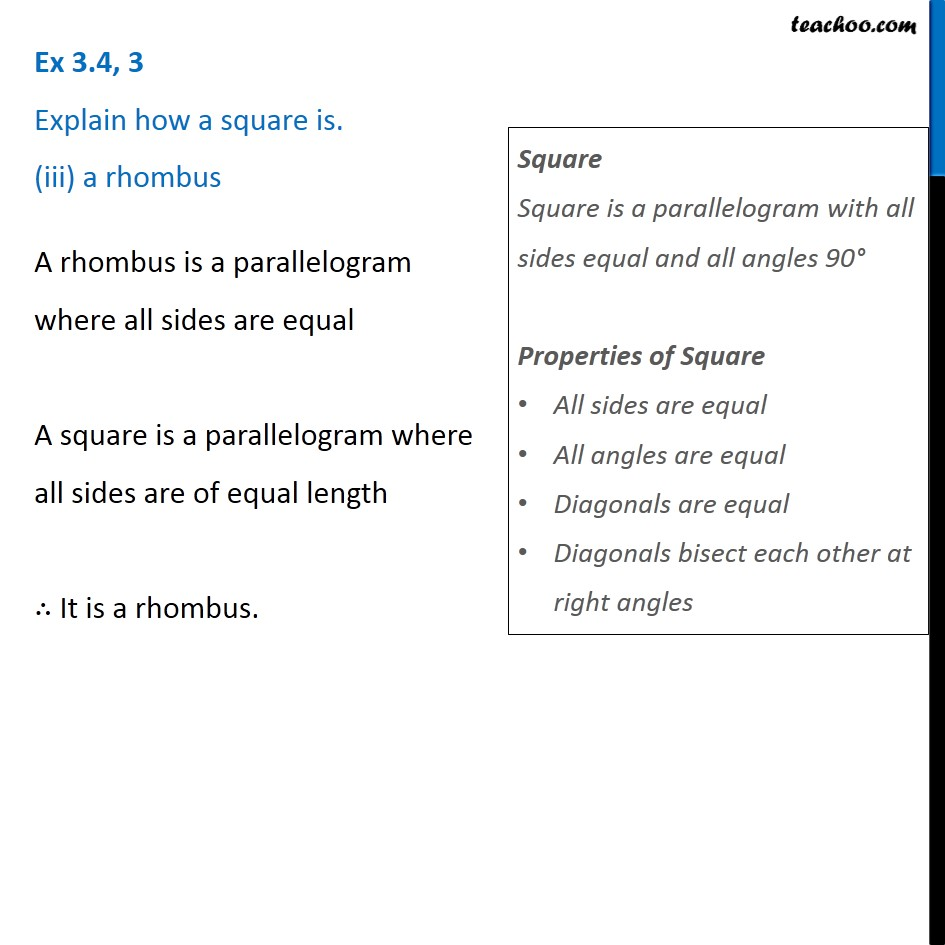Ex 3.4

Chapter 3 Class 8 Understanding Quadrilaterals
Serial order wiseGet live Maths 1-on-1 Classs - Class 6 to 12

### Transcript

Ex 3.4, 3 Explain how a square is. (iii) a rhombus A rhombus is a parallelogram where all sides are equal A square is a parallelogram where all sides are of equal length ∴ It is a rhombus. Square Square is a parallelogram with all sides equal and all angles 90° Properties of Square All sides are equal All angles are equal Diagonals are equal Diagonals bisect each other at right angles